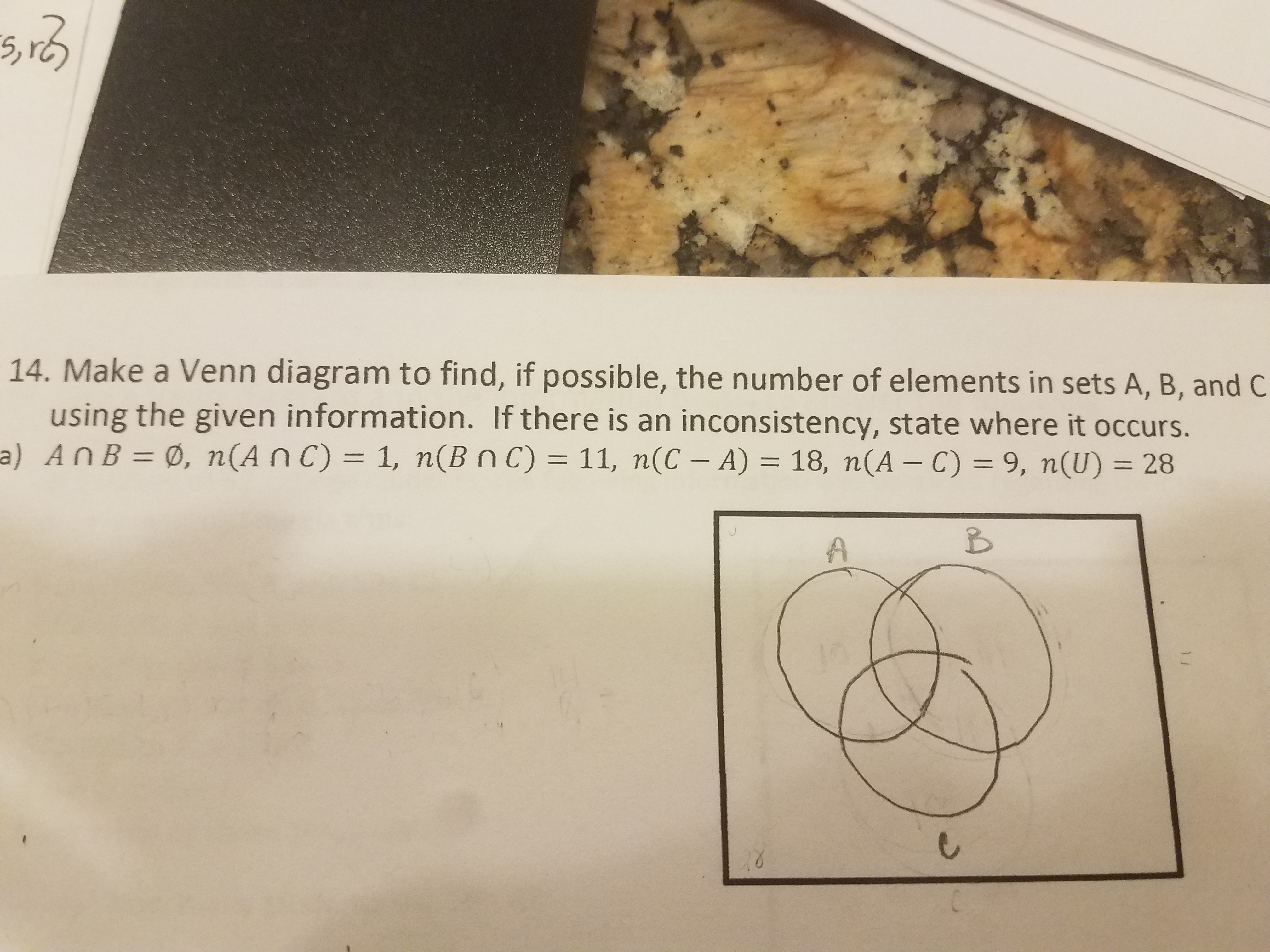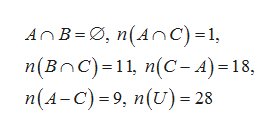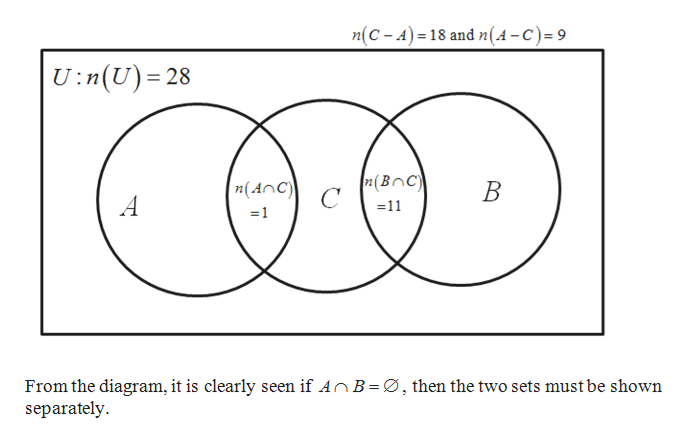14. Make a Venn diagram to find, if possible, the number of elements in sets A, B, and Cusing the given information. If there is an inconsistency, state where it occurs.a) AnB = Ø, n(An C) = 1, n(Bn c) 11, n(C - A) = 18, n(A - C) = 9, n(U) = 28A

Question

I dont know where to start.help_outlineImage Transcriptionclose14. Make a Venn diagram to find, if possible, the number of elements in sets A, B, and C using the given information. If there is an inconsistency, state where it occurs. a) AnB = Ø, n(An C) = 1, n(Bn c) 11, n(C - A) = 18, n(A - C) = 9, n(U) = 28 A fullscreen
Step 1

The given information ishelp_outlineImage TranscriptioncloseAnB , n(AnC)=1, n(Bnc)=11, n(C-A)-18 n(A-C)9, n(U)28 fullscreen
Step 2

With the given details, the Venn diagram is obtained ashelp_outlineImage Transcriptionclosen(C-A) 18 and n(4-C)=9 U:n(U) 28 72(BOC) n(AnC C B 11 A =1 From the diagram, it is clearly seen if AnB =Ø, then the two sets must be shown separately fullscreen
Step 3

Here, we don’t ...

Want to see the full answer?

See Solution

Want to see this answer and more?

Our solutions are written by experts, many with advanced degrees, and available 24/7

See Solution
Tagged in

Math